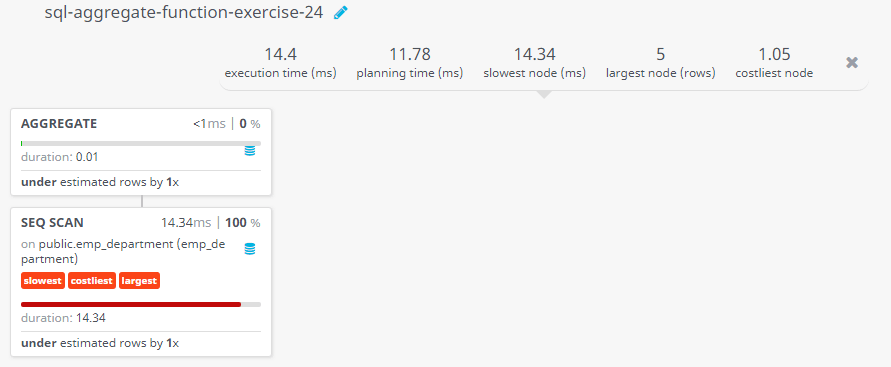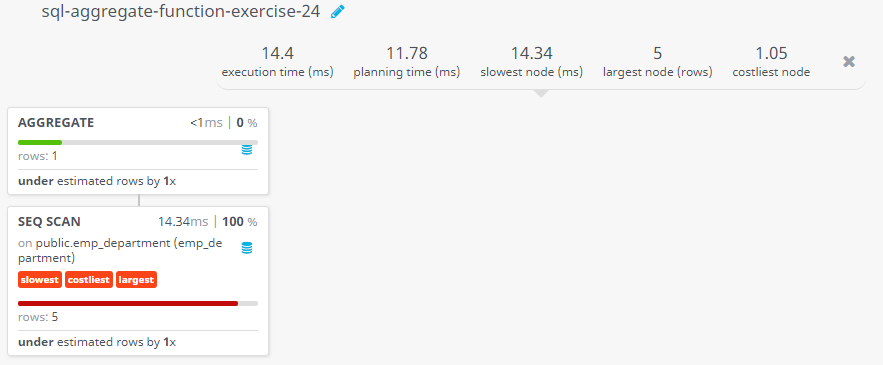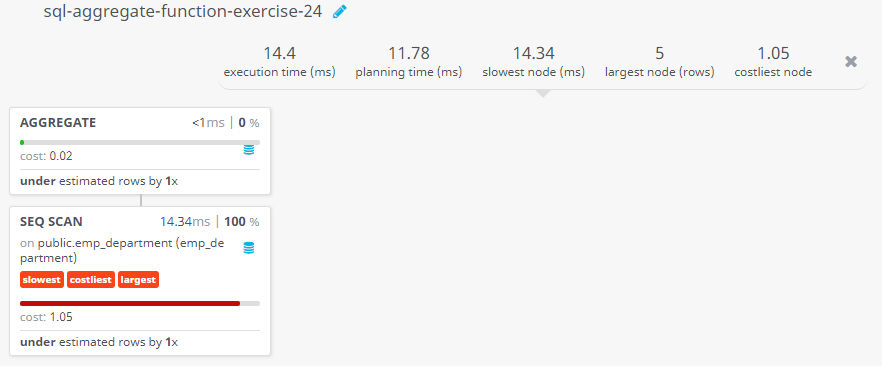﻿ SQL: Calculate the total allotment for all departments

# SQL Exercises: Calculate the total allotment for all departments

## SQL Aggregate Functions: Exercise-24 with Solution

From the following table, write a SQL query to compute the sum of the allotment amount of all departments. Return sum of the allotment amount.

Sample table: emp_department

Sample Solution:

``````SELECT SUM(dpt_allotment)
FROM emp_department;
``````

Output of the Query:

```sum
450000
```

Code Explanation:

The query in SQL retrieves the sum of the values in the "dpt_allotment" column from the 'emp_department' table. The result will be a single value representing the total sum of the "dpt_allotment" column.

Relational Algebra Expression:Relational Algebra Tree:## Query Visualization:

Duration:Rows:Cost:Have another way to solve this solution? Contribute your code (and comments) through Disqus.

Previous SQL Exercise: Compute the average price for unique companies.
Next SQL Exercise: Find out the number of employees in each department.

What is the difficulty level of this exercise?

Test your Programming skills with w3resource's quiz.

﻿

## SQL: Tips of the Day

What is the best way to paginate results in SQL Server?

```SELECT  *
FROM    ( SELECT    ROW_NUMBER() OVER ( ORDER BY OrderDate ) AS RowNum, *
FROM      Orders
WHERE     OrderDate >= '1980-01-01'
) AS RowConstrainedResult
WHERE   RowNum >= 1
AND RowNum < 20
ORDER BY RowNum
```

Database: SQL Server

Ref: https://bit.ly/3MGrNlk

We are closing our Disqus commenting system for some maintenanace issues. You may write to us at reach[at]yahoo[dot]com or visit us at Facebook